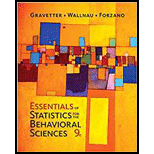# Explain why honesty is a hypothetical construct instead of a concrete variable. Describe how honesty might be measured and defined using an operational definition.### Essentials of Statistics for The B...

9th Edition
Frederick J Gravetter + 2 others
Publisher: Cengage Learning
ISBN: 9781337098120### Essentials of Statistics for The B...

9th Edition
Frederick J Gravetter + 2 others
Publisher: Cengage Learning
ISBN: 9781337098120

#### Solutions

Chapter
Section
Chapter 1, Problem 5P
Textbook Problem

## Expert Solution

### Want to see the full answer?

Check out a sample textbook solution.See solution

### Want to see this answer and more?

Experts are waiting 24/7 to provide step-by-step solutions in as fast as 30 minutes!*

See Solution

*Response times vary by subject and question complexity. Median response time is 34 minutes and may be longer for new subjects.

Find more solutions based on key concepts
Show solutions
Evaluate the expression sin Exercises 116. 323

Finite Mathematics and Applied Calculus (MindTap Course List)

Factoring Completely Factor the expression completely. 110. r2 6rs + 9s2

Precalculus: Mathematics for Calculus (Standalone Book)

Verify by differentiation that the formula is correct. tan2xdx=tanxx+C

Single Variable Calculus: Early Transcendentals, Volume I

In Exercises 47-52, find and simplify f(a+h)f(a)h(h0) for each function. 51. f(x)=1x

Applied Calculus for the Managerial, Life, and Social Sciences: A Brief Approach

For each pair of vectors, find UV. U=2i+9j,V=3ij

Trigonometry (MindTap Course List)

True or False: If , then converge absolutely.

Study Guide for Stewart's Single Variable Calculus: Early Transcendentals, 8th

True or False: For f(x, y) = x2(x + y), x and y are independent variables.

Study Guide for Stewart's Multivariable Calculus, 8th

21. A U.S. Senate Judiciary Committee report showed the number of homicides in each state. In Indiana. Ohio, an...

Modern Business Statistics with Microsoft Office Excel (with XLSTAT Education Edition Printed Access Card) (MindTap Course List)

Solve the integral equation f(t)=et+et0tef()d.

A First Course in Differential Equations with Modeling Applications (MindTap Course List)

Finding a Window Find an appropriate window set up will show a good graph of y=x2+10x2+5 with a horizontal span...

Functions and Change: A Modeling Approach to College Algebra (MindTap Course List)